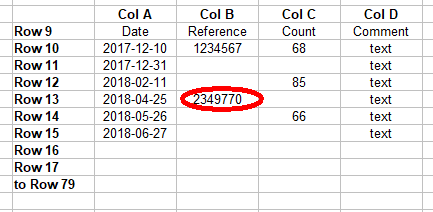# How do I find the last entry in a column that may contain many blanks (Solved)

I wish to easily find the last value in a column that may contain blank entries. I am allowing up to 70 rows of data.

Column A will contain the Date of an entry, but not every other column will have an entry on that date.
I would like to find a way to determine what row contains the last entry in Column B, so I can select the corresponding date in Column A

In the accompanying image -The range is A10:D79. Column A will contain consecutive rows of data (presently to Row 15) the last entry in Column B is in Row 13. How do I find the row number of that value?I want the row number of the range A10:D79 that would correspond to that circled entry (relative row 4) so I can use it, along with the offset (+9) to determine the actual row number (13) in that sheet. Then I can use the indirect address function to extract the required value for the date on that row.

Other related questions seem to assume that ALL columns in any row are filled, and do not seem to address my particular need where there are many blank rows that are irregularly spaced in the target column.

=MATCH(1E+018,MyRange,1) ; where my range is the range B9:B79 provides the offset of 4. You can use the offset function as you planned.

=MATCH(1E+018,B:B,1) ; where the range uses the entire column will yeild the row of the actual cell, 13. No offset function required.

Both of these are a bit hacky because they assume that you can provide a number for the search criterion that is larger than any number that will exist in column B. I just entered a bunch of 9’s.

The Formula extendet to return the relativ Date from Column A:.

``=INDEX(A9:A79,MATCH(1E+018,B9:B79,1))``

Or: =INDIRECT(“a”&MATCH(1E+018,B:B,1))
Lots of ways to get the same result:)